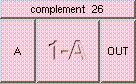COMPLEMENTTHE BOX'S MAIN HELP: Result is 1-A. This is the complement of A. A may be a float, point or color. The result will be the same data type. A should lie in the range [0,1]. Vectors should be normalized. A (input): The value to be reversed. This may be a float, point, or color. The default is float. Inputs should lie in the range [0,1]. Vector should be normalized. OUT (output): Result is 1-A. This is the complement of A. The result will be the same data type as A. Output will be in the range [0,1].

<-- BACK TO Math